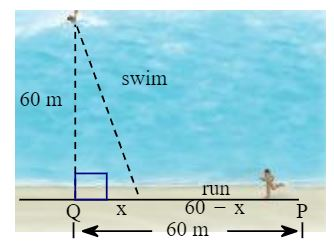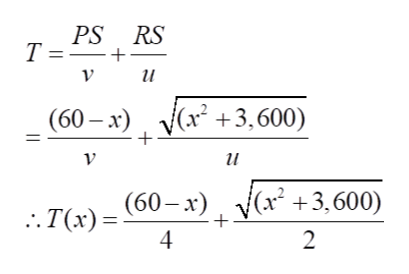Question
67 views

You must get from a point P on the straight shore of a lake to a stranded swimmer who is 60 meters from a point Q, on the shore that is 60 m from you (see figure).  If you can swim at a speed of 2 m/s and run at a speed of 4 m/s, at what point along the shore, x meters from Q, should you stop running and start swimming if you want to reach the swimmer in the minimum time?

a. Given the above write the function T that gives the travel time as a function of x, where 0 <= x <= 60.help_outlineImage Transcriptioncloseswim 60 m I run 60-X 60 m P X fullscreen
check_circle

Step 1

Let, "u" be the velocity at which you can swim and "v" be the velocity at which you can run.

u = 2 m / s

Please see the diagram on the white board. I have marked two more points "R" and "S". Please look at the triangle QRS.

v = 4 m / sStep 2

RS = distance that you have to swim through.

Triangle QRS is a right angled triangle. Hence, make use of Pythagoras theorm to write the following equation:

RS2 = QS2 + QR2

Or, RS2 = x2 + 602 = x2 + 3,600

Hence, RS = (x2 + 3,600)1/2

Step 3

Total travel time T = Time taken while running + time taken while swimming = Distance to ru...help_outlineImage TranscriptionclosePS RS Т%3D+ и (60 — х) a +3,600) и x3,600) . Т(х) - (60— х) 2 fullscreen

### Want to see the full answer?

See Solution

#### Want to see this answer and more?

Solutions are written by subject experts who are available 24/7. Questions are typically answered within 1 hour.*

See Solution
*Response times may vary by subject and question.
Tagged in

### Functions